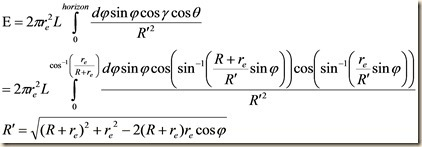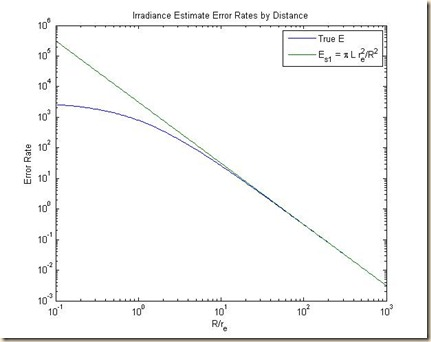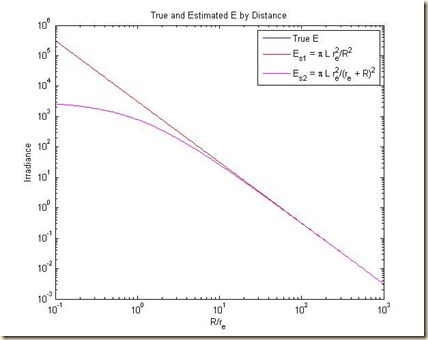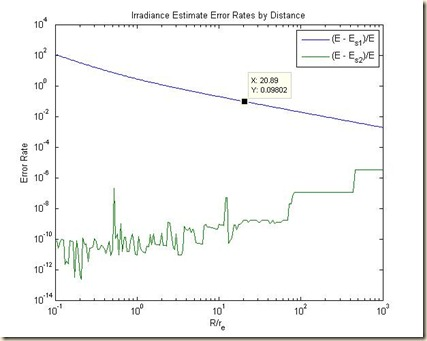## Thursday, July 26, 2012

### Radiometric Error Evaluation, Part V: Results

In the last post, I promised to evaluate the integral:This is only partially true.  I have no idea how to solve an equation like this analytically, although I know the correct answer.  What I can do, however, is solve it numerically, setting our constant radiance = 1000, the radius of our emitting sphere = 1:

function [R,E]=intE()
L = 1000;
re = 1;
l1 = 0; % Sensor nadir
figure;
for i = 1:200,
R = 10.^(-1+.02*i);
l2 = acos(re./(R + re)); % Limit of field of view;
end;
Rprime = sqrt((R + re)^2 + re^2 - 2*(R+re)*(re).*cos(phi));
gamma = asin(((R + re)./Rprime).*sin(phi));
theta = asin((re./Rprime).*sin(phi));

E = (2*pi*L*re^2./Rprime.^2.*sin(phi).*cos(gamma).*cos(theta));
end
end

I graphed the value of E vs. R, along with the value of our estimate from two lessons ago:The estimate appears to be pretty good for R >> reBut before I graph the error, I decided to add to the estimate E = π L re2/R2 the estimate E = π L re2/(R +re)2:Well, how about that.  It turns out E = π L re2/(R +re)2: was so good an estimate it completely overwrote the true value across all values of R.

Let’s plot the error.By the looks of it, the error of Es2 (taken as an absolute value to graph on a log scale) is pretty much limited to accumulated random rounding errors, although these errors might be getting larger the farther away my receiving aperture is, and the smaller the emitting surface appears.

Basically, this is saying that for purposes of analysis,